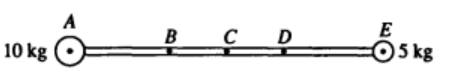# Problem: 10. A 5-kilogram sphere is connected to a 10-kilogram sphere by a rigid rod of negligible mass, as shown above. The sphere-rod combination can be pivoted about an axis that is perpendicular to the plane of the page and that passes through one of the five lettered points. Through which point should the axis pass for the moment of inertia of the sphere-rod combination about this axis to be greatest? (A) A (B) B (C) C (D) D (E) E

###### FREE Expert Solution

The spheres are equivalent to point masses.

Moment of inertia of a point mass:

For a collection of point masses:

82% (85 ratings)###### Problem Details10. A 5-kilogram sphere is connected to a 10-kilogram sphere by a rigid rod of negligible mass, as shown above. The sphere-rod combination can be pivoted about an axis that is perpendicular to the plane of the page and that passes through one of the five lettered points. Through which point should the axis pass for the moment of inertia of the sphere-rod combination about this axis to be greatest?

(A) A

(B) B

(C) C

(D) D

(E) E